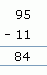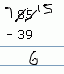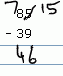Name: ___________________Date:___________________

 Email us to get an instant 20% discount on highly effective K-12 Math & English kwizNET Programs!

### Grade 2 - Mathematics1.40 Subtracting - Two Digits

Subtraction without borrow
Method:
1. Start from the ones place
2. Subtract the numbers in the ones place
3. Subtract the numbers in the tens place

Example:

 Question Step 1 Step 2Subtraction with borrow
Method:
1. Look at the numbers in the ones place.
2. If the number on top is more than the number at the bottom. Borrow 1 from the tens place.
3. Decrease 1 from the tens place and add 10 to ones place.
4. Now perform subtraction for the ones place.
5. Look at the numbers in the tens place
6. Perform similar subtraction for the tens place
Example:

 Question Step 1 Step 2The ones digits, 5 is less than 9, therefore borrow 1 from tens place that is from 8.
Decrease 1 from 8 and add 10 to ones place to become 15.
Subtract 15 and 9 to get 6.
Subtract 7 and 3 to get 4.

Directions: Answer the following questions. Also write at least 5 examples of your own for subtraction without borrow and subtraction with borrow.Name: ___________________Date:___________________

### Grade 2 - Mathematics1.40 Subtracting - Two Digits

 Question 1: 81 - 73

 Question 2: 18 - 4

 Question 3: 44 - 41

 Question 4: 64 - 12

 Question 5: 97 - 86

Question 6: This question is available to subscribers only!

Question 7: This question is available to subscribers only!

Question 8: This question is available to subscribers only!

Question 9: This question is available to subscribers only!

Question 10: This question is available to subscribers only!

#### Subscription to kwizNET Learning System costs less than \$1 per month & offers the following benefits:

• Unrestricted access to grade appropriate lessons, quizzes, & printable worksheets
• Instant scoring of online quizzes
• Progress tracking and award certificates to keep your student motivated
• Unlimited practice with auto-generated 'WIZ MATH' quizzes